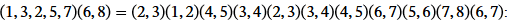• I
Gold Member
Question: In defining adjacent transpositions in a permutation as swaps between neighbors, is one referring to the original set or to the last result before the transposition is applied? I clarify with an example.
Suppose one assumes a beginning ordered set of <1,2,3>
It is clear that (1,2) (2,3), and (1,3) are the adjacent transpositions for <1,2,3>
However, if I compose them (2,3)(1,2), I first apply the transposition (1,2) to <1,2,3> I now have <2,1,3> and now 2 and 3 are no longer neighbors. So is (2,3) still considered an adjacent transposition?
According the the definitions I find on the Internet, it appears that the answer is yes, but this goes contrary to the intuition of sapping neighbors at each step.
Thanks.

Initial statement: (1,3) - end points - adjacent. After trans. (2,3) - end points - not adjacent. Doesnt look right.

Gold Member
Initial statement: (1,3) - end points - adjacent. After trans. (2,3) - end points - not adjacent. Doesnt look right.
Thanks, mathman. I am not sure which part doesn't look right to you. I picked a short example, but perhaps a longer example would be appropriate. (I can give the source if desired.)Homework Helper
Gold Member
2022 Award
So is (2,3) still considered an adjacent transposition?
According the the definitions I find on the Internet, it appears that the answer is yes, but this goes contrary to the intuition of swapping neighbors at each step.
Thanks.
The numbers in the transposition are fixed dummy variables. ##(2,3)## always means the same thing: swap the second and third elements in the permutation. It does not mean "swap the numbers 2 and 3, wherever they may be". You can look at ##(2, 3)## as the mapping:
$$(2, 3): (x, y, z) \rightarrow (x, z, y)$$

•